# Mathematics For Middle School

This quiz contains lots of different questions of mathematics including various topics like number theory, decimals, integers, fractions, measurement, probability and so on, covering the course content of mathematics of Grade 7. Various types of questions are included in the quiz to make it more interactive and many real-life examples are used to let students feel the importance of mathematics.

Start Quiz

What is the Greatest Common Factor(GCF) of 12 and 16?

##4##

4

At a family reunion, each of Sara's aunts and uncles is getting photographed once. The aunts are taking pictures in groups of 5 and the uncles are taking pictures in groups of 7. If Sara has the same total number of aunts and uncles, what is the minimum number of uncles that Ashley must-have?

##35## uncles

35

How do you write 4.66 x 10^5 in standard form?

##466000##

466000

Type the missing integer.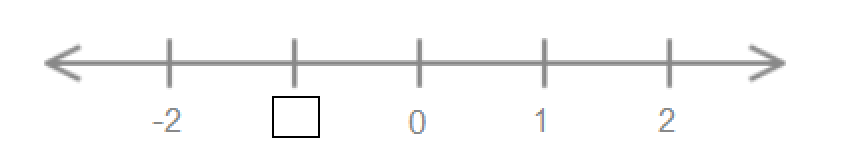##-1##

-1

Which fraction is equivalent to 6/7?

3/4

12/14

7/6

11/21

Select all the rational numbers from the given options.

12/5

√3

√16

3.2

Solve for u in the proportion.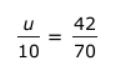5

6

7

8

Are the ratios 4:5 and 20:25 equivalent?

yes

no

Fill in the blanks with the correct symbol.

76% ##>## 52%

< > > =

In an exam Ashley secured 332 marks. If she secured 83 % makes, find the maximum marks.

300

500

350

400

What kind of sequence is this?

12, 15, 18, 21, 24 …..

Arithmetic

Geometric

Both

Neither

Is 2/7 a rational number?

yes

no

A chocolate bar is 3/4 of an inch long. If it is divided into pieces that are 3/8 of an inch long, then how many pieces is that?

2

3

4

5

Simplify the expression.

8k + 2(3k +5)

8k + 10

14k + 10

14k + 5

Find the value of b for the given angles of the triangle.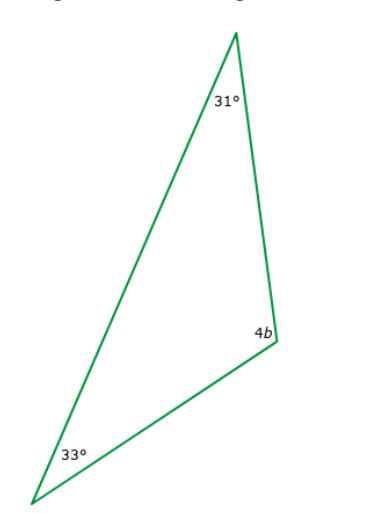b= ##29##

29

Two figures are said to be congruent, if they have exactly the same:

length and width

shape and size

area

perimeter

Which of the given angles are interior alternate angles?(Select all that apply)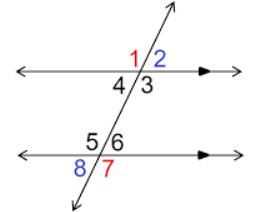Angle 5 and Angle 2

Angle 3 and Angle 5

Angle 4 and Angle 6

Angle 3 and Angle 6

100 students were asked what their primary mode of transport for getting to school was. The results of this survey are recorded and the results are recorded in the form of a bar graph given below.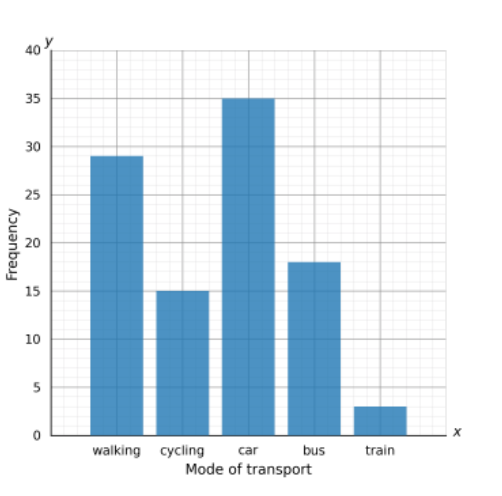Which mode of transport is used the most by the students?

walking

cycling

car

bus

In a throw of two dice, the probability of getting a sum of exactly 4 is-

1/12

1/2

1/4

1/8

What is the probability of drawing an ace card from a pack of 52 cards?

1/13

4/13

12/52

9/52

Quiz/Test Summary
Title: Mathematics For Middle School
Questions: 20
Contributed by: